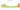# F

## CFP Franc

#### how has the value of the currency changed in the last year?## USD 1 = XPF 113.05

How much is10 US dollars worth inCFP francs?
At the current exchange rate, 10 US dollars is worth 1,130.46 CFP francs
How much is50 US dollars worth inCFP francs?
At the current exchange rate, 50 US dollars is worth 5,652.32 CFP francs
How much is100 US dollars worth inCFP francs?
At the current exchange rate, 100 US dollars is worth 11,304.64 CFP francs
How much is500 US dollars worth inCFP francs?
At the current exchange rate, 500 US dollars is worth 56,523.18 CFP francs
How much is2,000 US dollars worth inCFP francs?
At the current exchange rate, 2,000 US dollars is worth 226,092.73 CFP francs
How much is10 US dollars worth inCFP francs?
At the current exchange rate, 10 US dollars is worth 1,130.46 CFP francs
How much is50 US dollars worth inCFP francs?
At the current exchange rate, 50 US dollars is worth 5,652.32 CFP francs
How much is100 US dollars worth inCFP francs?
At the current exchange rate, 100 US dollars is worth 11,304.64 CFP francs
How much is500 US dollars worth inCFP francs?
At the current exchange rate, 500 US dollars is worth 56,523.18 CFP francs
How much is2,000 US dollars worth inCFP francs?
At the current exchange rate, 2,000 US dollars is worth 226,092.73 CFP francs